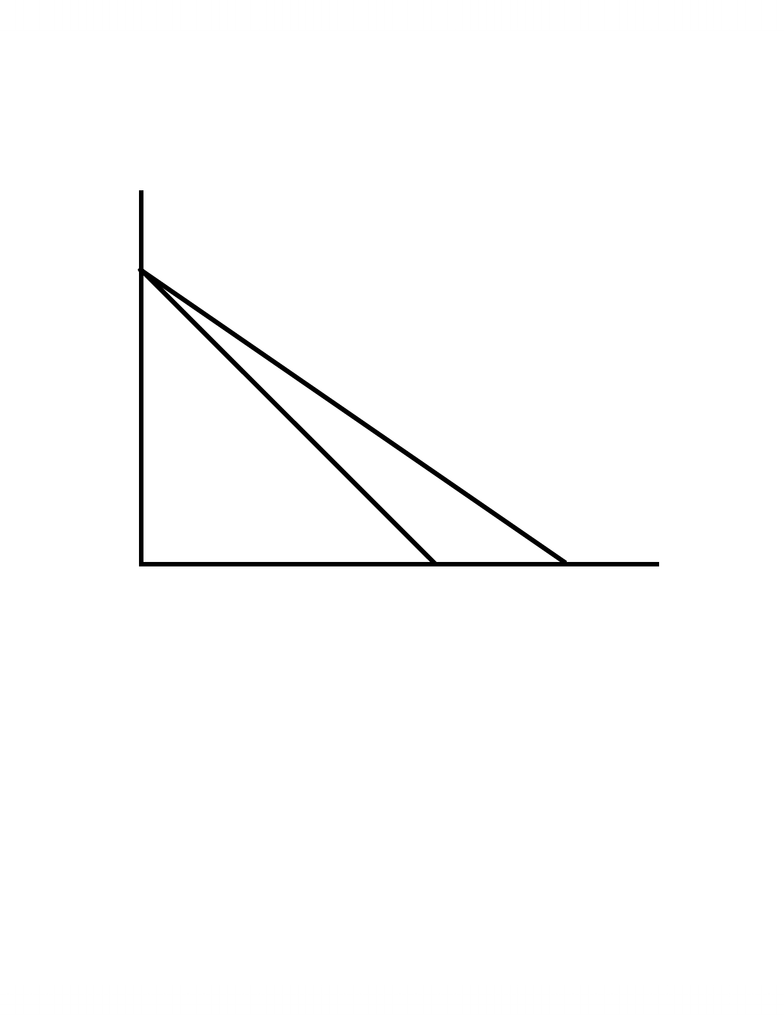Class Notes (1,100,000)
CA (630,000)
UTM (20,000)
ECO (800)
A (10)
Lecture

ECO206Y5 Lecture Notes - Ceteris Paribus, Ordinal Utility, Tangent

Department
Economics
Course Code
ECO206Y5
Professor
A

This preview shows pages 1-3. to view the full 9 pages of the document.Preference Theory and Derivation of Demand
CONSUMER CHOICE THEORY (DEMAND)
BUDGET CONTRAINTS:
- Suppose that the consumer (household) consumes only two goods (X and Y).
Given the Prices of the two goods (PX, PY) and the consumer’s income (m), the possible
quantities purchasable by the consumer (Xo, Yo) are constrained by
PXXo + PYYo m
e.g., Suppose that a student has a budget for coffee and milk of \$100/month. If the price of Coffee
(X say) is \$1/cup and the price of Milk (Y) is \$2/litre, the budget constraint is
\$1*QC + \$2*QM \$100
Note: We can define one of the goods (Y say) as a composite commodity, representing all goods
other than X.
Budget Line:
Definition: The maximum combination of two commodities purchasable by a consumer given the
prices of the two commodities and the consumer’s money income
Rearranging the budget equation for the assumption that all income is spent gives the Budget line
Yo = m/PY – PX/PY *X0
e.g. The budget line for Coffee and Milk with Milk as the Y commodity is
QM = \$100/\$2 – \$1/\$2*QC = 50 – 0.5QC
Since the Opportunity Cost of X = -dY/dX
(Recall: Opportunity Cost = the best foregone option)
Opportunity Cost of X = -dY/dX = PX/PY (= -slope of the budget line)
(=> dY = -PX/PY *dX)
- 1 -

Only pages 1-3 are available for preview. Some parts have been intentionally blurred.Preference Theory and Derivation of Demand
e.g. The opportunity cost of a unit of Coffee = -(-0.5) = 0.5 Milk
i.e., one unit of Coffee costs ½ litre of Milk
Q
Y
Q
X
m/P
Xo
m/P
Y
Budget Line Constraint
slope = -P
Xo
/P
Y
m/P
X1
'
slope = -P
X1
/P
Y
- The diagram above shows the budget line for m, PXo, PY, and the budget line that ensues given
a fall in the price of X to PX1. A change in one of the prices causes a rotation of the budget
line around the intercept of the commodity whose price is unchanged
Note: A change in Income causes a parallel shift in the budget line: an increase in income shifts it
out and an increase in income shifts it in.
PREFERENCES AND INDIFFERENCES CURVES
For any two consumption bundles (X0, Y0) and (X1, Y1), define an individual’s preference
ranking of each of the two bundles by
1. (X1, Y1) > (X0, Y0) means the individual prefers (X1, Y1) to (X0, Y0)
- 2 -

Only pages 1-3 are available for preview. Some parts have been intentionally blurred.Preference Theory and Derivation of Demand
2. (X1, Y1) ~ (X0, Y0) means the individual is indifferent between (X1, Y1) and (X0, Y0)
Preference Assumptions
1. Completeness
For every pair of consumption bundles (X1, Y1) and (X0, Y0), (X1, Y1) > (X0, Y0) or (X0, Y0) >
(X1, Y1) or (X1, Y1) ~ (X0, Y0)
i.e. The consumer prefers one or other bundle or is indifferent between them. In short the
consumer does not make contradictory choices.
2. Transitivity
(X1, Y1) (X0, Y0) and (X0, Y0) (X2, Y2) => (X1, Y1) (X2, Y2)
(where means either preferred to or indifferent to)
3. Non-Satiation (More is always preferred to Less)
If both X1 or Y1 are at least equal to X0 or Y0 respectively and at least one of X1 or Y1 is a
greater amount than X1 or Y1 respectively, then
(X1, Y1) (X0, Y0)
This assumption is sometimes called the monotonicity of preferences
4. Convex
The less one has of a good, the more one requires of the other good in exchange to remain
indifferent.
[Assumption 1 follows from the assumption that economic agents are rational
Assumption 2 is actually an assumption since transitivity need not necessarily hold. Indeed,
transitivity for groups of individuals often does not hold.
Assumption 3 and 4 are actually assumptions about well-behaved indifference curves.
- 3 -
You're Reading a Preview

Unlock to view full version